Problem title
Difficulty
Avg time to solve

Closest Leaf To Given Node In Binary Tree
Moderate
15 mins
Max Stack
Easy
15 mins
Count triplets in a sorted doubly linked list whose sum is equal to a given value x
Moderate
15 mins
Binary Tree Zigzag Traversal
Easy
15 mins
Count elements in all subtrees
Easy
10 mins
Jumping Numbers
Hard
10 mins
Ninja And Candies
Moderate
15 mins
Z Algorithm
Hard
15 mins
Is Graph Tree?
Easy
15 mins
Minimum Swaps To Convert Binary Tree Into BST
Moderate
20 mins10

# Preorder Traversal

Difficulty: EASY

Problem Statement

#### You have been given a Binary Tree of 'N' nodes, where the nodes have integer values. Your task is to find the Pre-Order traversal of the given binary tree.

##### For example :
``````For the given binary tree:
``````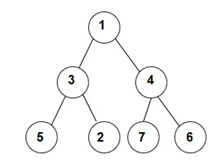``````The Preorder traversal will be [1, 3, 5, 2, 4, 7, 6].
``````
##### Input Format :
``````The first line contains an integer 'T' which denotes the number of test cases.

The first line of each test case contains elements of the tree in the level order form. The line consists of values of nodes separated by a single space. In case a node is null, we take -1 in its place.
``````
##### Example :
``````The input for the tree is depicted in the below image:
``````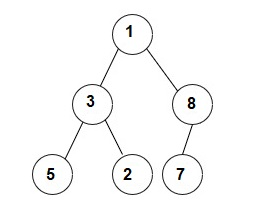``````1 3 8 5 2 7 -1 -1 -1 -1 -1 -1 -1
``````

#### Explanation :

``````Level 1 :
The root node of the tree is 1

Level 2 :
Left child of 1 = 3
Right child of 1 = 8

Level 3 :
Left child of 3 = 5
Right child of 3 = 2
Left child of 8 =7
Right child of 8 =  null (-1)

Level 4 :
Left child of 5 = null (-1)
Right child of 5 = null (-1)
Left child of 2 = null (-1)
Right child of 2 = null (-1)
Left child of 7 = null (-1)
Right child of 7 = null (-1)

1
3 8
5 2 7 -1
-1 -1 -1 -1 -1 -1
``````
##### Note :
``````1. The first not-null node(of the previous level) is treated as the parent of the first two nodes of the current level. The second not-null node (of the previous level) is treated as the parent node for the next two nodes of the current level and so on.

2. The input ends when all nodes at the last level are null(-1).

3. The above format was just to provide clarity on how the input is formed for a given tree. The sequence will be put together in a single line separated by a single space. Hence, for the above-depicted tree, the input will be given as:

1 3 8 5 2 7 -1 -1 -1 -1 -1 -1 -1
``````
##### Output Format :
``````For each test case, return a vector containing the Pre-Order traversal of a given binary tree.

The first and only line of output of each test case prints 'N' single space-separated integers denoting the node's values in Pre-Order traversal.
``````
##### Note :
``````You don't need to print anything, it has already been taken care of. Just implement the given function.
``````
##### Constraints :
``````1 <= T <= 10
0 <= N <= 3000
0 <= data <= 10^9

Where 'data' denotes the node value of the binary tree nodes.

Time limit: 1 sec
``````
##### Sample Input 1 :
``````2
1 2 3 -1 -1 -1  6 -1 -1
1 2 3 -1 -1 -1 -1
``````
##### Sample Output 1 :
``````1 2 3 6
1 2 3
``````
##### Explanation of Sample Output 1 :
`````` In test case 1, the given binary tree is shown below:
``````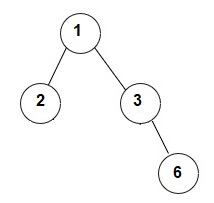``````Preorder traversal of given tree = [1, 2, 3, 6]

In test case 2, the given binary tree is shown below:
``````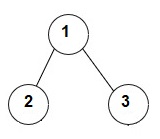``````Preorder traversal of given tree = [1, 2, 3]
``````
##### Sample Input 2 :
``````2
1 -1 -1
1 2 4 5 3 -1 -1 -1 -1 -1 -1
``````
##### Sample Output 2 :
``````1
1 2 5 3 4
``````
##### Explanation of Sample Output 2 :
``````In test case 1, there is only one node, so Pre-Order traversal will be only .

In test case 2, the given binary tree is shown below:
``````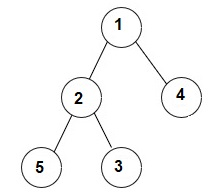``````Preorder traversal of given tree = [1, 2, 5, 3, 4]
``````Console